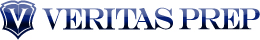# How To Use Remainders

- Nov 26, 10:00 AM Comments 

We're betting that you haven’t used a remainder in years. Remainders in division are pretty much just placeholders for kids who haven’t yet learned about mixed numbers or decimals yet; as soon as you learn what to do with the remainder, you tend to never use a  remainder again…until you take the GMAT.

Consider the problem 11/3. 3*3 is 9, but then there are 2 left over that won’t divide evenly. So in this case, 11/3 = 3 remainder 2.

But by now you’ve figured out that you can divide that remaining 2 by 3, either as a mixed number:

3 2/3

Or as a decimal:

2/3 = .6667, so 11/3 = 3.6667

As with many concepts on the GMAT, your ability to use all three ways of dealing with numbers that are not evenly divisible will be important — you’ll need to stay flexible to see problems from multiple angles, and often times the GMAT will test the conceptual (e.g. remainders) applications of these problems more so than it will test the “calculational” (decimals), plug-and-chug methods to which you’ve become accustomed.

Sometimes the GMAT will simply test the concept of a remainder. Consider the question:

If 13,333 – n is divisible by 11, and 0 <> n is the remainder of that problem

Now, because we don’t care about the quotient of this problem — the question solely asks for the remainder, we simply need to determine what’s left over when we divide 13333 by 11. The fastest way to do that is to simply subtract large multiples of 11 from 13333 to see what’s left. We can do this because of the rule a (x + y) = ax + ay. If we’re trying to divide a number like, say 51 by 3, we can break apart that 51 into 21 and 30 so that we have 7*3 + 10*3, which equals 3(7+10) = 3*17 = 51. In this case, we can break apart 13,333 by taking off large multiples of 11:

13333 – 11000 = 2333
2333 – 2200 = 133
133 – 121 = 12
12 – 11 = 1

The remainder, then, is 1.

Another way that the GMAT will test remainders goes back to the concept at the beginning of this post. The remainder of a division problem is what you would just divide back into the problem to determine the decimals:

25/4 = 6 remainder 1.
Divide that 1 back by 4 to get .25, so the answer is 6.25. The remainder provides the data after the decimal point, and the quotient gives you the number to the left of the decimal point.

Consider this problem (which appears courtesy of GMAC):

When positive integer x is divided by positive integer y, the remainder is 9. If x/y = 96.12, what is the value of y?

(A) 96
(B) 75
(C) 48
(D) 25
(E) 12

Going back to the concept of the remainder, the remainder of 9 is what will give us that .12 after the decimal place. The answer to the division problem x/y is either:

96 remainder 9
or
96.12

Therefore, when the remainder of 9 is divided back over y, we get .12. Mathematically, this means that:

9/y = .12
9 = .12y
900 = 12y (multiplying both sides by 100 to eliminate the need to deal with decimals can make calculation much easier!)
900/12 = y
300/4 = y (factor out the 3)
75 = y

Understanding the concept of remainders — what they are, how they’re calculated, and how they can be turned into fractions/decimals — can be quite useful on this test, so as you study take a glance back at your elementary school years and hopefully your knowledge of remainders will remain.

Ready to sign up for a GMAT course? Enroll through GMAT Club and save up to \$180 (use discount code GMATC10)! Take a look at our course options in some of our most popular cities: New York, Chicago and Los Angeles .  Save 50% off our our new live online  Essentials Course being offered the weekend of December 4th and 5th - use code GMATC50!